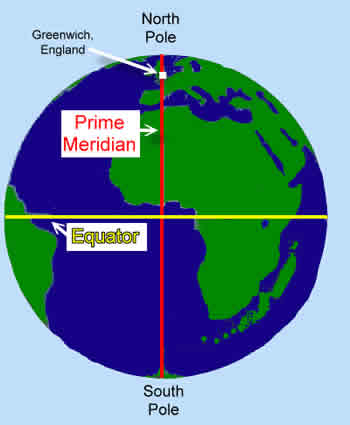# Difference between Equator and Prime Meridian

##### Key Difference: The equator is the imaginary circle that draws a line between the Northern hemisphere and Southern hemisphere. It is the point from where the latitude is calculated. In other words, the equator is at 0° latitude. The prime meridian is the vertical line that marks the zero degree longitude measurement on the globe of Earth and intersects the latitude lines, which are horizontal.The term equator is derived from the Latin phrase, 'circulus aequator diei et noctis', meaning ‘circle equalizing day and night’. The equator is the imaginary circle that draws a line between the Northern hemisphere and Southern hemisphere. It is the point from where the latitude is calculated. In other words, the equator is at 0° latitude. It is one of the main imaginary latitude circles , which is actually based on the relationship between the Earth’s axis of rotation.

In simple terms, equator is the imaginary circle, which cuts the spherical Earth exactly in the middle, i.e. in two halves. At latitude 0° (the equator), the day length will be approximately 12 hours. Parts on the equator have a constant 12 hours of day light all year round.

The term prime meridian is an imaginary line from north pole to south pole that draws a vertical line, which intersects the latitude lines that are horizontal. It basically divides the Earth into two hemispheres, i.e. Eastern hemisphere and Western hemisphere. Since one uses directions from East and West from a defined prime meridian, then it is called as the two hemispheres.

In 1884, at an International Conference the prime meridian was officially established as zero degrees longitude. Greenwich was selected as the prime meridian by a vote of twenty-two in favor, one against (Haiti), and two abstentions (France and Brazil). By establishing the prime meridian and 0° longitude in Greenwich, the world was then divided into 24 time zones (since the earth takes 24 hours to revolve on its axis) and thus each time zone was established in every fifteen degrees of longitude, for a total of 360° in a circle. The term Prime Meridian is also written as PM; and it is basically referred to as time.

Comparison between Equator and Prime Meridian:

 Equator Prime Meridian Description The equator is the imaginary circle, which cuts the spherical Earth exactly in the middle, i.e. in two halves. The prime meridian is the vertical line that marks the zero degree longitude measurement on the globe of Earth and intersects the latitude lines that are horizontal. Imaginary Line Line Line Horizontal Vertical 0° The equator is at 0° latitude. The Prime Meridian is at 0° longitude. Separates Northern hemisphere and Southern hemisphere Eastern hemisphere and Western hemisphere

Image Courtesy: primaryhomeworkhelp.co.uk